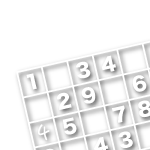TOP

# Creating a Sudoku program 3

## Sudoku solver program

It is necessary to validate the problem as a solvable one.
It is meaningless if I create numerous unsolvable problems.
So, I created a program that solves problems.

## First, enter the numbers in the blank numbers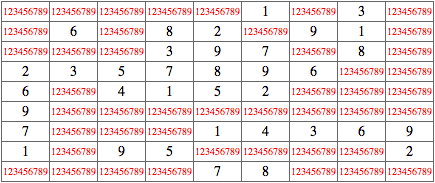First, it enters 123456789 in the blank boxes. Then it deletes invalid numbers and if only 1 remains at the end, it becomes the final number.
Programs can do these tedious tasks accurately as many times as possible, so it’s quite convenient.

## Deleting invalid numbers

Since there are numbers that are already confirmed, I need to delete them from the potential numbers.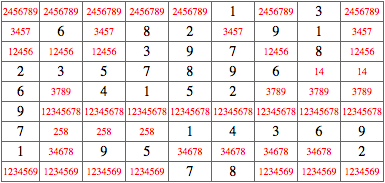In the diagram above, the numbers that do not go in the horizontal direction are deleted. The potential numbers for the 2nd column decrease significantly, leaving only 3457.Here, the numbers that do not go in the vertical direction are deleted. It’s not so crowded.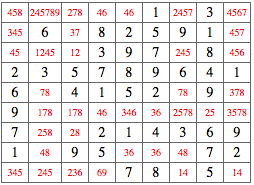Then it deletes the numbers that cannot go in the 9 boxes. There are 9 3x3 boxes. Now we have new confirmed boxes.

## Confirm sole candidate numbers

In the diagram above, 2578 is in column 6. The 5 in here is not in any other candidates for this column.This means the only box that 5 is a candidate is 2578. So this box becomes a 5. It then repeats this process in the horizontal direction.It then repeats this process in the vertical direction.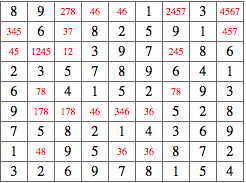It repeats this whole process for the 9 3x3 boxes.

## Delete the numbers in pair from the candidate

In the diagram above, we see 2 boxes with 46 in the first column. These 2 pairs will end up with 4,6 or 6,4. We don’t know which is which yet, but we know that 4 and 6 will not go in the other boxes.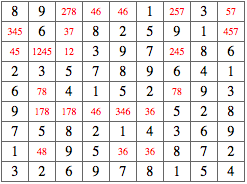So, it deletes 46 from the other boxes and on the top right, we are left with 57.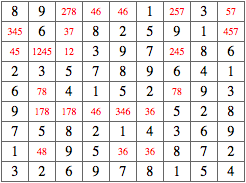It repeats this process in the vertical direction.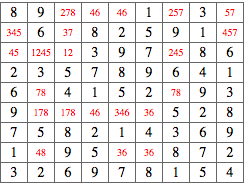It repeats this process in the 9 3x3 boxes.

### Even for the box with a trio

The pair number rule applies for trios, so the same process is used.

### When there are 4 pairs in the square

This is pretty rare, but sometimes we get 4 pairs that line up on the square. In such cases, it removes them from the candidate as well.

### This process is then repeated

If the program cannot solve the problem at this point, it goes back to the beginning and starts removing potential numbers again, since we have more confirmed numbers now. A normal problem can be solved with this program, so if it passes this test, it becomes a valid question.

### Unsolvable problems

When a problem is unsolvable, no matter how many times the process is repeated, if a problem cannot be solved, it cannot be solved. So the program must be able to detect that.
To be more specific, if the status below and after is the same, it gives up and moves on to the next one.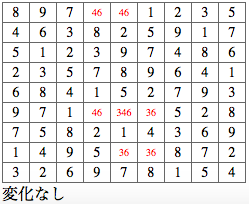By the way, this problem stopped changing here and became unsolvable. At a closer look,
46
643
36

64
463
36
are both answers that fulfill the puzzle, so it has more than 1 answer.

Next: Creating problems with fewer clues